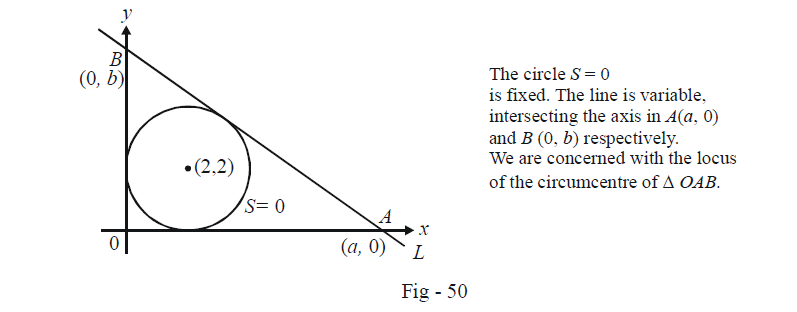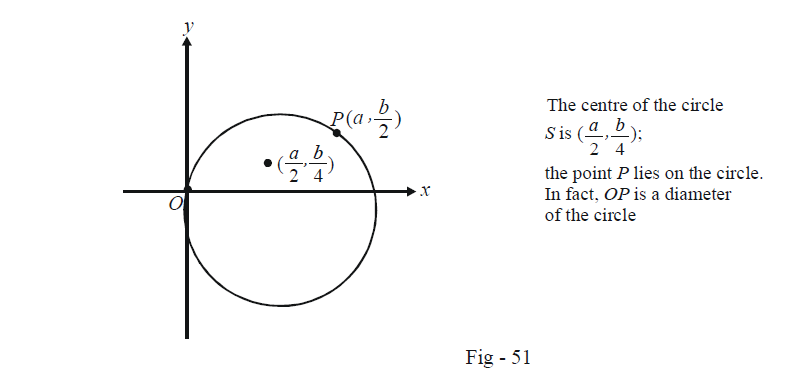# Circles Set-1

Go back to  'SOLVED EXAMPLES'

Example – 1

The circle $${x^2} + {y^2} - 4x - 4y + 4 = 0$$ is inscribed in a triangle which has two of its sides along the co-ordinate axes. If the locus of the circumcentre of the triangle is

$x + y - xy + k\sqrt {{x^2} + {y^2}} = 0$

find the value of k.

Solution: The situation is described clearly in the figure below:The equation of L is, using the intercept form,

$L:\frac{x}{a} + \frac{y}{b} = 1$

The distance of the centre of S, i.e. (2, 2) from L must equal the radius of S which is 2. Thus,

\frac{{\left| {\frac{2}{a} + \frac{2}{b} - 1} \right|}}{{\sqrt {\frac{1}{{{a^2}}} + \frac{1}{{{b^2}}}} }} = 2\,\,\,\,\,\,\,\,\,\,\,\,\,\,\,\,\,\,\left\{ \begin{align}&{\text{We now use the fact that }}\\&L{\text{(2, 2) is negative since}}\\&{\text{(2, 2) and the origin lie }}\\&{\text{on the same side of }}L{\text{ and}}\\&L{\text{(0, 0) is negative }}\end{align} \right\}

$\Rightarrow \quad 2a + 2b - ab + 2\sqrt {{a^2} + {b^2}} = 0\,\,\,\,\,\,\,\,\,\,\,\,\,\,\,\,...\left( 1 \right)$

From pure geometric considerations, the circumcentre C of $$\Delta OAB$$ lies on AB and is in fact, the  mid-point of AB.

Thus,

$C \equiv \left( {\frac{a}{2},\frac{b}{2}} \right)$

Slightly manipulating (1), we obtain

$\frac{a}{2} + \frac{b}{2} - \left( {\frac{a}{2}} \right)\left( {\frac{b}{2}} \right) + \sqrt {{{\left( {\frac{a}{2}} \right)}^2} + {{\left( {\frac{b}{2}} \right)}^2}} = 0\,\,\,\,\,\,\,\,\,\,\,\,\,\,\,\,\,\,\,...(2)$

The locus of \begin{align}\left( {\frac{a}{2},\frac{b}{2}} \right)\end{align} is given by (2). Using $$(x,y)$$ instead of \begin{align}\left( {\frac{a}{2},\frac{b}{2}}\right),\end{align} we obtain

$x + y - xy + \sqrt {{x^2} + {y^2}} = 0\,\,\,\,\,\,\,\,\,\,\,\,\,\,\,\,\,\,\,\,\,...(3)$

Upon comparing (3) with the locus specified in the question, we obtain $$k = 1.$$

Example – 2

Consider a curve $$a{x^2} + 2hxy + b{y^2} = 1$$ and a point P not on the curve. A line drawn from P intersects the curve at points Q and R. If the product $$PQ \cdot PR$$ is independent of the slope of the line, then show that the curve is a circle.

Solution: Since distances are involved from a fixed point, it would be a good idea to use the polar form of the line to write the co-ordinates of Q and R.

Let P be $$({x_1},{y_1})$$ and let $$\theta$$ denote the slope of the variable line. For any point $$(x,y)$$  lying on this line at a distance r from P, we have

\begin{align}&\,\,\,\,\,\,\,\,\,\,\,\,\,\,\,\,\,\,\frac{{x - {x_1}}}{{\cos \theta }} = \frac{{y - {y_1}}}{{\sin \theta }} = r\\&\quad\Rightarrow \quad x = {x_1} + r\cos \theta \,\,;\,\,y = {y_1} + r\sin \theta \end{align}

If $$(x,y)$$  lies on the given curve, it must satisfy the equation of the curve :

\begin{align}&a{({x_1} + r\cos \theta )^2} + b{({y_1} + r\sin \theta )^2} + 2h({x_1} + r\cos \theta )({y_1} + r\sin \theta ) = 1\\& \Rightarrow \quad \{ a{\cos ^2}\theta + b{\sin ^2}\theta + h\sin 2\theta \} {r^2} + 2\{ a{x_1}\cos \theta + b{y_1}\sin \theta + h({x_1}\cos \theta \\&\qquad\qquad \qquad\qquad + {y_1}\sin \theta )\} r + ax_1^2 + by_1^2 + 2h{x_1}{y_1} - 1 = 0\qquad\qquad \quad...(1)\end{align}

This quadratic has two roots in r, say $${r_1}$$ and $${r_2}$$, which will actually correspond to $$PQ$$ and $$PR$$ since Q and R lie on the curve. Thus, $$PQ \cdot PR$$ being independent of $${\rm{\theta }}$$ means that $${r_1}{r_2}$$ for (1) is independent of $${\rm{\theta }}$$ i.e.

\begin{align} & \frac{ax_{1}^{2}+by_{1}^{2}+2h{{x}_{1}}{{y}_{1}}-1}{a{{\cos }^{2}}\text{ }\!\!\theta\!\!\text{ }+b{{\sin }^{2}}\text{ }\!\!\theta\!\!\text{ }+h\sin 2\text{ }\!\!\theta\!\!\text{ }}\,\,\text{is independent of}\,\,\theta \\ \\ & \Rightarrow \quad \frac{ax_{1}^{2}+by_{1}^{2}+2h{{x}_{1}}{{y}_{1}}-1}{\left( \frac{a+b}{2} \right)+\left( \frac{a-b}{2} \right)\cos 2\text{ }\!\!\theta\!\!\text{ }+h\sin 2\text{ }\!\!\theta\!\!\text{ }}\,\,\text{is independent of}\,\,\theta \\ \\ & \left\{ \text{The denominator was obtained in this form by writing }{{\cos }^{2}}\theta \text{ as }\frac{1+\cos 2\theta }{2}\text{ and }{{\sin }^{2}}\theta \text{ as }\frac{1-\cos 2\theta }{2} \right\} \\ & \Rightarrow \quad \frac{ax_{1}^{2}+by_{1}^{2}+2h{{x}_{1}}{{y}_{1}}-1}{\left( \frac{a+b}{2} \right)+\sqrt{{{\left( \frac{a-b}{2} \right)}^{2}}+{{h}^{2}}}\{\sin (2\theta +\phi \}}\,\,\text{is independent of}\,\,\theta \\ \\ & \left\{ \text{The denominator was obtained by combining the two trignometric terms}\text{.}\tan \phi \,\,\text{is }\left( \frac{a-b}{2h} \right)\text{ } \right\} \\ & \Rightarrow \quad \text{This is only possible when}{{\left( \frac{a-b}{2} \right)}^{2}}+{{h}^{2}}=0 \\ & \Rightarrow \quad a=b\,\,\,and\,\,\,h=0 \\ \end{align}

Thus, the equation of the given curve reduces to

$$a{x^2} + a{y^2} = 1$$

which is clearly the equation of a circle.

Example – 3

The equation of a circle is $$S:2x(x - a) + y(2y - b) = 0$$ where $$a,\,\,b \ne 0.$$  Find the condition on a and b if two chords each bisected by the x-axis, can be drawn to the circle from the point \begin{align}P\left( {a,\frac{b}{2}} \right).\end{align}

Solution: Observe that the centre of S is \begin{align}\left( {\frac{a}{2},\frac{b}{4}} \right)\end{align} and P lies on the circle:Let us consider a chord $$PQ$$ of the circle bisected at the x-axis, say, at the point $$(h,0).$$ We can write the equation of $$PQ$$ as :

$T(h,0) = S(h,0)$

where $$S(x,y)$$ is

\begin{align} &{x^2} + {y^2} - ax - \frac{b}{2}y = 0\\ \Rightarrow \qquad & hx - \frac{a}{2}(x + h) - \frac{b}{4}(y + 0) = {h^2} - ah\\ \Rightarrow \qquad & \left( {h - \frac{a}{2}} \right)x - \frac{b}{4}y + \left( {\frac{{ah}}{2} - {h^2}} \right) = 0 \qquad\qquad ...(1) \end{align}

Since this chord passes through \begin{align}P\left( {a,\frac{b}{2}} \right),\end{align}  the co-ordinates of (P) must satisfy (1). Thus,

\begin{align}&\qquad\; \left( {h - \frac{a}{2}} \right)a - \frac{b}{4}\left( {\frac{b}{2}} \right) + \frac{{ah}}{2} - {h^2} = 0\\& \Rightarrow \quad \frac{{3ah}}{2} - \frac{{{a^2}}}{2} - \frac{{{b^2}}}{8} - {h^2} = 0\\& \Rightarrow \quad {h^2} - \frac{{3ah}}{2} + \frac{{{a^2}}}{2} + \frac{{{b^2}}}{8} = 0\,\,\,\,\,\,\,\,\,\,\,\,\,\,\,\,\,\,\,\,\,\,\,\,\,\,\,\,...(2)\end{align}

We want to have two such possible chords PQ bisected at the x-axis. Thus, we must have two distinct values of h which can happen if the discriminant of (2) is positive. Thus,

\begin{align}&\,\,\,\,\,\,\,\,\,\,\,\,\,\,\,\,\frac{{9{a^2}}}{4} > 4\left( {\frac{{{a^2}}}{2} + \frac{{{b^2}}}{8}} \right)\\ &\Rightarrow \qquad {a^2} > 2{b^2}\end{align}

This is the required condition that a and b must satisfy.

Learn from the best math teachers and top your exams

• Live one on one classroom and doubt clearing
• Practice worksheets in and after class for conceptual clarity
• Personalized curriculum to keep up with school